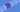# JavaScript - Functions

## Creating Functions

Functions are reusable blocks of code that allow users to easily rerun a procedure. These will help us reduce repeated code and give us a convenient way to run tasks numerous times. Let's start with an example.

``````function formatCurrency(amount) {
return `\$\${amount}`
}

formatCurrency(100)``````

There are a few things going on here. First, notice how we use the `function` keyword to tell the compiler we want to declare a function. Let's look at some pseudocode to see the formula of a function.

``````function [nameOfFunction]([listOfParameters]) {
return [returnValue]
}``````

So, we can declare a function by typing `function` followed by the name, then we add `()` with an parameters we want, and finally end with `{}` our familiar `block` of code. Inside the block, we can use the `return` keyword (but we don't have to), to return a value to the caller.

## Using Functions

Our function above returns a string that formats the number passed in. In the example above, the function creates a variable called `amount` which holds the number passed in by the caller. Let's see how to call our function.

``````fromatCurrency(100) // 100 is stored in amount. This returns \$100
fromatCurrency(200) // 200 is stored in amount. This returns \$200
fromatCurrency(30000) // 30000 is stored in amount. This returns \$30000``````

Our formatter is not the best, but you can see how we can easily repeat the formatting of the number by simply calling our function. Also note, that if we update the formatter to handle more logic (like adding a comma for numbers > 1000), we only have to change it in one place.

GoTea - KoalaTea